### A Comparison of the Performance of Flexible Functional Forms for Use in Applied General Equilibrium Analysis

#### Carlo Perroni

Department of Economics
University of Warwick
Coventry CV4 7AL, U.K.

and

#### Thomas F. Rutherford

Department of Economics

January 1996

* Research supported by the Electric Power Research Institute and the GAMS General Equilibrium Research Fund.

#### Abstract

This paper presents a procedure for testing the global properties of functional forms which recognizes their specific role in economic equilibrium modeling. This procedure is employed to investigate the regularity and the third-order curvature properties of three widely used flexible functional forms, the Translog, the Generalized Leontief and the Normalized Quadratic functional forms. We contrast the results from these functions with a globally regular flexible form, the Non-separable Nested Constant- Elasticity-of-Substitution functional form. Our results indicate that inherently regular representations have clear advantages for equilibrium analysis.

#### 1 Introduction

This paper explores the global properties of four flexible functional forms in order to assess their comparative performance and suitability for use in applied equilibrium modeling exercises. Flexible functional forms (FFFs) have been widely adopted for empirical econometric work, but there have been relatively few instances in which they have been employed to model production and consumption choices in applied general equilibrium models (Hudson and Jorgenson (1984); Jorgenson and Slesnick (1985); Reister and Edmonds (1981)). Few modellers have adopted FFFs for the reason that, in spite of their superior local ap- proximations, they generally exhibit poor global properties.

Research on the use of FFFs in equilibrium models has been scant. Caves and Christensen (1980) built a number of examples to compare the regular regions of the Translog (TL) (Christensen, Jorgenson and Lau (1971)) and Generalized Leontief (GL) (Diewert (1971)) functional forms. Performance was found to depend on the initial specification of second order curvature conditions, with the TL being preferable when the cross Allen-Uzawa elasticities of substitution (AUES) are close to unity (Allen (1938), Uzawa (1962)), and the GL being a good choice for cross AUESs close to zero. Reister and Edmonds (1981) analyzed the effects of replacing a Constant-Elasticity-of-Substitution (CES) specification (Uzawa (1962)) with a TL form in a simple equilibrium model and found substantial differences in their out-of-benchmark behaviour. Comparing the global properties of the TL and GL functions, Despotakis (1986) concluded that the law of change of the AUES is different for these two functions, and he noted that these differences can have important consequences for equilibrium analysis.

While in econometric modeling, functional forms are used to estimate the local characteristics of technologies or preference orderings from a given set of observations, in applied general equilibrium analysis functional forms are used as a global representation of technologies and preferences. Here, the information available to the modeller for the specification of technologies and preferences is typically local, i.e., limited to a small region of production or consumption sets. This local information is extrapolated to the full domain of the modeling exercise by specifying production or utility functions that are locally consistent with that information, an approach which is often referred to as "calibration" (Shoven and Whalley (1992)).

In applied general equilibrium applications, the global properties of functional forms become important. Lack of global regularity, which may not be crucial for econometric estimation, may cause numerical solution methods to fail even when functions are well behaved at the equilibrium point. Furthermore, when analyzing discrete policy changes, third-order curvature properties, which are of little consequence for the purposes of econometric estimation, can crucially affect estimates of welfare impacts, both in total and at the margin.

The evaluation criteria we employ in this paper reflect the specific role of functional forms in applied general equilibrium modeling vis a vis econometric applications. We develop a testing procedure which investigates both the global regularity properties and the third-order curvature properties of functional forms. Summary measures of global regularity are obtained by computing the area of the region in price space over which a cost function is well behaved (i.e., non-negative, monotonic, and convex in prices). We also obtain summary measures of global third-order curvature properties by computing the area of the region over which the function remains close, in a sense to be defined by the modeller, to a given local specification of curvature conditions.

These tests are applied to four FFFs: the Translog (Christensen, Jorgenson and Lau (1971)), the Generalized Leontief (Diewert (1971)), the Normalized Quadratic (NQ) (Diewert and Wales (1987)), and the Nonseparable Nested CES (NNCES) (Perroni and Rutherford (1995)). We choose to focus on the three-input case both because it represents a good compromise between simplicity and generality and also because of its practical relevance (e.g., in the modeling of substitution possibilities among labour, capital and energy inputs). Our tests uncover fundamental differences in the global behaviour of different functional forms and lead us to conclude that convex technologies are better suited for equilibrium modeling than traditional FFFs.

#### 2 Evaluating the global properties of functional forms

Our discussion will be focused on production technologies with N inputs, one output and constant returns-to-scale. In most applications, a sufficient and convenient representation of such technologies is given by a continuous unit cost function, C(p,1), where p denotes the input price vector and 1 is the production level. This will be hereafter referred to simply as C(p) or C. The first derivatives of C, C_i, represent conditional input demands (Shephard's Lemma); these are homogeneous of degree zero in prices and, by Euler's Theorem, satisfy the adding-up condition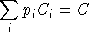. The matrix of partial second derivatives [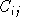] (the Hessian) is homogeneous of degree -1 in prices and satisfies the Cournot aggregation condition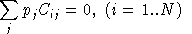.

In the following, we will normalize prices so that price vectors lie in the unit simplex: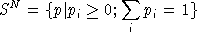. We will also express conditional demands in terms of input value shares: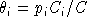and the Hessian of the cost function in terms of Allen-Uzawa elasticities of substitution, which are defined as. The AUES is a dimensionless index of curvature, and is thus scale-invariant. The Euler condition for the AUES matrix has the form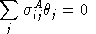.

Regularity

A cost function is regular (well-behaved) at a point p if its value C(p) is non-negative, its first-derivatives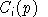(which correspond to input demands) are non-negative

(monotonicity), and if the Hessian [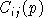] is negative semidefinite (concavity, a sufficient condition for the choice of inputs to minimize cost). Monotonicity implies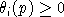and negative semidefiniteness of [] implies the same for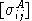.

We will refer to the range over which a function maintains monotonicity as the Monotonic Domain (MD). This can be expressed as

2.1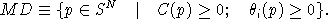The range over which a function maintains concavity will be referred to as its the Concave Domain (CD):

2.2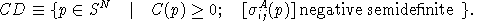The region of the price simplex over which a cost function is regular, which Despotakis (1986) termed the Outer Domain, is then simply

2.3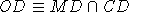.

Third-order curature properties

Despotakis (1986) defines the Inner Domain (ID) of a cost function as the region of the price simplex where the function provides a good approximation to the "true" technology. In applied modeling exercises, however, the information available to the modeller is typically limited to the calibration point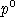, and the "true" technology is unknown. In the absence of global information on the technology to be approximated, the modeller must adopt, explicitly or implicitly, certain assumptions concerning the out-of-benchmark characteristics of functions, on the basis of the local information available. For example, when choosing a CES representation (Uzawa (1962)), a modeller implicitly assumes that when moving away from the benchmark point the first and second derivatives of the cost function change in such a way as to ensure constancy of all cross AUESs. This corresponds to a specific set of conjectures about the third- order curvature properties of the cost function.

To make the notion of Inner Domain operational when only local information is available, we can employ a distance function Z:

2.5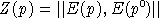where E(p) is a vector of curvature measures (e.g., elasticities) at p, and E() represents the corresponding values at the benchmark point. The definition of E(.) is left to the discretion of the modeller.

Assuming that Z(p) has been defined, the Inner Domain can be defined as the region of the unit simplex where the value of Z is less than or equal to a pre-specified tolerance level, i.e.,

2.4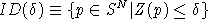.

The choice of a particular curvature index implies certain assumptions about the global characteristics of the cost function. For example, if the modeller believes that the "true" cost function exhibits constant value shares, then E(p) will be chosen to represent a vector of value shares (in which case the best choice of functional form would naturally be a Cobb-Douglas cost function). In the following, we will restrict our discussion to a few second-order curvature indexes that have proposed in the literature.

A well known dimensionless index of second-order curvature is the compensated price elasticity (CPE), which is defined as

2.5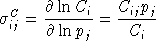.

A related measure of second-order curvature is the AUES, which has been already discussed. This can also be written as

2.6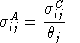.

The AUES is a one-input-one-price elasticity of substitution (Mundlak (1968)), since, as (2.6) makes clear, it measures the responsiveness of the compensated demand for one input to a change in one input price. In contrast, the Morishima elasticity of substitution (MES) (Morishima (1967)) constitutes a two-input- one-price elasticity measure, being defined as

2.7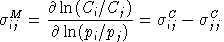.

Note that, in general, the MES is not symmetric, i.e.,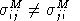.

A third measure of curvature is the represented by the class of two-input-two-price elasticities of substitution, which take the form: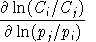. One such index is the shadow elasticity of substitution (SES) (Frenger (1985)), which is defined as

2.8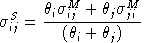.

When technologies are of the CES type,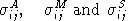are all identical, but they are generally different otherwise.

In our tests, we define the distance function Z as a weighted sum of the square deviations from the benchmark elasticity of substitution matrix, where weights are chosen to be equal to the combined share of inputs i and j in total cost:

2.9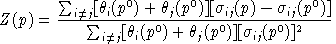.

We employ four different versions of the above norm, respectively based on the CPE (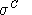), AUES (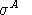), MES (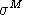), and SES (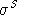).

#### 3 Experimental design

We can obtain synthetic indexes, AMD and ACD, respectively for the Monotonic and Concave Domains, by measuring the volume of these regions as a proportion of the volume of the unit price simplex, i.e.,

3.1 AMD = Area(MD) / Area(S);

3.2 ACD = Area(CD) / Area(S).

For the Inner Domain we must also specify a tolerance level:

3.3 AID( ) = Area[ID( )] / Area(S).

The testing procedure is as follows. The function under investigation is calibrated at a benchmark pointto a given specification of derivatives up to the second order. The properties of the function (monotonicity, concavity, and Z(p) values) are then systematically evaluated over a triangular grid on the price simplex containing 325 points. The resulting discrete mapping is then contoured to derive piecewise-linear approximations of the various domains. Finally, the approximated contour sets are used to compute the areas AMD, AD, and AID( ).

In the tests reported here, we choose p0 to be the center of the unit simplex and we consider two configurations of benchmark value shares: a symmetric configuration with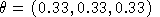and an asymmetric configuration with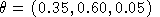. For each configuration of value shares, we examine a number of different benchmark configurations of second-order curvature conditions belonging to the regular region, i.e., the set of benchmark cross AUES configurations that are compatible with local concavity of the cost function.

Because of symmetry and homogeneity, only H = N (N - 1) / 2 elements of the matrix [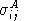] are independent, which implies that we can ignore the diagonal terms. Thus, the regular region Q is a subset of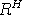, bounded by N - 1 conditions for negative semidefiniteness of the AUES matrix. Q is a convex set, since a convex combination of two negative semidefinite matrices is also negative semidefinite. Moreover, Q is a cone, since multiplication of a negative semidefinite matrix by a positive scalar results in a negative semidefinite matrix.

The latter property enables us to characterize the geometry of the regular region analyzing only the image of a projection of Q in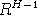. For this purpose we choose the following projection: the AUES matrix is divided by its largest positive off-diagonal element, so that the maximum off-diagonal element of the resulting matrix is always unity. In our testing, we focus on the case N = 3 and, without loss of generality, assume that the element (1,2) is the largest cross AUES. Then, the regular region Q lies in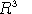and the image of the projection lies in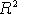, bounded by the following constraints ([] denotes the normalized AUES matrix):

3.4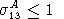3.5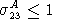3.6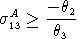3.7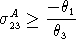3.8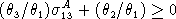3.9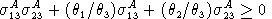The first two constraints follow from normalization. The remaining four are the sign constraints on the first and second principal minors for negative semidefiniteness.

Our testing procedure has been employed to explore four "slices" through Q, those representing AUES matrices with maximum off-diagonal values respectively equal to 1/2, 1, 2 and 4. For each of these sections we examined a uniform grid of AUES configurations with between 47 and 52 points depending on the benchmark value shares. The resulting measures AMD, ACD, and AID( ) (taking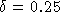to define the Inner Domain) are averaged over all sample points.

#### 4. Test results

We tested four different functional forms: TL, GL, NQ and NNCES. The TL and GL forms have been chosen because they are the best known among FFFs. The NQ form is included because it is globally concave (although it can lose monotonicity). The NNCES form is flexible and globally regular, and belongs to a family of functional forms that have been widely employed in the applied general equilibrium literature. All four functional forms, and the formulae used for parameter calibration, are described in the appendix.

Results of our Outer Domain calculations are summarized in Table 1, which reports average measures of MD, CD, and OD as a percentage of the price simplex for different specifications of maximum cross AUES.

#### Table 1: Average measured Outer Domain dimensions

% of the price simplex

Symmetric Value Shares:View Table with Detailed Results

```               Monotonic Domain    Concave Domain       Outer Domain
--------------     --------------     --------------
sigma12 =    .5   1   2   4     .5   1   2   4     .5   1   2   4
--------------     --------------     --------------
TL           71  82  81  32     27  62  73  60     25  61  65  27
GL          100  99  69  30     93  93  93  93     93  92  63  27
NQ          100 100  73  33    100 100 100 100    100 100  72  31
NNCES       100 100 100 100    100 100 100 100    100 100 100 100
```

Asymmetric Value Shares:```               Monotonic Domain    Concave Domain       Outer Domain
--------------     --------------     --------------
sigma12 =    .5   1   2   4     .5   1   2   4     .5   1   2   4
--------------     --------------     --------------
TL           69  86  72  28     46  71  76  64     41  70  61  25
GL          100  98  61  25     95  95  94  92     94  92  57  24
NQ          100  99  67  27    100 100 100 100    100  99  66  26
NNCES       100 100 100 100    100 100 100 100    100 100 100 100
```

Our findings are consistent with earlier studies of the global properties of the TL and GL functional forms. The TL is prone to loss of concavity away from the benchmark point whenever the benchmark elasticities depart from unity. The GL tends to lose monotonicity as benchmark elasticities increase. The NQ remains concave over the entire domain but, tends to lose monotonicity at higher elasticities, as does the GL. The Outer Domain for the NQ (as well as the TL and GL) falls below 50% of the price simplex when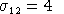and benhmark value shares are equal. When benchmark shares are asymmetric and, the TL, GL and NQ Outer Domain shrinks to roughly 1/4 of the price simplex. In contrast, the NNCES form is globally regular.

Table 2 reports corresponding results of Inner Domain calculations based respectively on the CPE, AUES, MES, and SES forms. The CPE based norm appears to be more volatile than the other measures, but the ranking of functional forms is consistent across different norms. The NQ performs poorly in all cases. In the symmetric case (upper panel), the TL form performs best for benchmark cross AUES values close to unity, and the GL form performs best for cross AUES values respectively close to zero. In the asymmetric cases the TL performs rather poorly, particularly for low cross AUES values. The NNCES is the most consistent performer.

#### Table 2: Average measured Inner Domain dimensions

% of the price simplex

Symmetric Value Shares:View Table with Detailed Results

```            Compensated         AUES           Morishima          Shadow
--------------   --------------   --------------   --------------
sigma12 =  .5   1   2   4   .5   1   2   4   .5   1   2   4   .5   1   2   4
--------------   --------------   --------------   --------------
TL          3  43  20   5    6  62  35   8   12  61  50  12   13  58  47  12
GL         25  40  17   4   67  59  22   6   81  75  30   8   83  76  29   8
NQ          4   4   3   1   11   9   6   3   21  12   6   2   20  14   6   2
NN         14  40  13   3   67  71  59  41   71  67  61  52   70  67  59  47
```

Asymmetric Value Shares:```            Compensated         AUES           Morishima          Shadow
--------------   --------------   --------------   --------------
sigma12 =  .5   1   2   4   .5   1   2   4   .5   1   2   4   .5   1   2   4
--------------   --------------   --------------   --------------
TL          7  63  18   4    9  59  36   9   16  66  44  12   13  57  43  12
GL         42  63  17   4   84  61  27   7   93  70  33   9   91  68  32   9
NQ          2   2   2   1    1   1   1   1    6   5   4   2   21  15   9   3
NN         35  78  20   4   66  74  68  50   92  90  83  73   88  87  83  66
```

Comparison of Tables 1 and 2 suggests that the Outer Domain and the Inner Domain of a cost function might be correlated. To test this conjecture formally, we have computed the correlation coefficient between the sizes of the Outer Domain and the AUES Inner Domain over the range of benchmark elasticity values (Table 3). These results confirm that the "instability" of cost functions is closely associated with loss of regularity.

#### Table 3: Correlation between the AUES Inner and Outer Domains

```                   Uniform  Shares               Nonuniform Shares

sigma12 =     .5     1     2     4           .5     1     2     4
----------------------         ----------------------
TL          0.92  0.92  0.85  0.90         0.96  0.97  0.93  0.77
GL          0.51  0.67  0.79  0.91         0.77  0.72  0.79  0.82
NQ           na    na   0.59  0.68         0.76  0.83  0.91  0.75
```

na: NQ is globally regular for these shares and assumed values, and the correlation is therefore undefined.

#### 5 Summary and conclusion

This paper has presented a procedure for testing the global properties of functional forms which explicitly recognizes their role in equilibrium modeling. We have used this procedure to test the regularity and third-order curvature properties of four flexible functional forms, and we found that the Translog, Generalized Leontief and Normalized Quadratic forms are all prone to loss of regularity, particularly when the benchmark cross- elasticities are large. Globally regular functions, like the NNCES, are better at preserving local calibration information over the domain of modeling exercises. For these reasons, we conclude that globally regular functions like the NNCES are better suited for equilibrium analysis.

Further research should investigate the global properties of alternative specifications of the NNCES, so to provide practitioners with some concrete guidance in the selection of nesting structure. A better understanding of the properties of the functional forms used in applied equilibrium exercises would improve transparency and ultimately contribute to users' understanding of model results.

University of Warwick

and

#### References

ALLEN, R.G.D. (1938) Mathematical Analysis for Economists, London: Macmillan.

CAVES, D.W. and L.R. CHRISTENSEN (1980), "Global Properties of Flexible Functional Forms," American Economic Review 70, 422- 432.

CHRISTENSEN, L.R., D.W. JORGENSON and L.J. LAU (1971), "Conjugate Duality and the Transcendental Logarithmic Production Function," Econometrica 39, 255-256.

DESPOTAKIS, K.A. (1986), "Economic Performance of Flexible Functional Forms: Implications for Equilibrium Modeling," European Economic Review 30, 1107-1143.

DIEWERT, W.E. (1971), "An Application of The Shephard Duality Theorem: A Generalized Leontief Production Function," Journal of Political Economy 79, 481-507.

DIEWERT, W.E. and T.J. WALES (1987), "Flexible Functional Forms and Global Curvature Conditions," Econometrica 55, 43-68.

FRENGER, P. (1985), "Using the Directional Shadow Elasticity of Substitution to Measure the Concavity of the Cost Function," Economics Letters 19, 171-175.

HUDSON, E.A. and D.W. JORGENSON (1974), "U.S. Energy Policy and Economic Growth, 1975-2000," Bell Journal of Economics and Management Science 5(2), 461-514.

JORGENSON, D.W. and D. SLESNICK (1985), "General Equilibrium Analysis of Economic Policy," in New Developments in Applied General Equilibrium Analysis (J. Piggott and J. Whalley, eds), Cambridge University Press.

MUNDLAK, Y. (1968), "Elasticities of Substitution and the Theory of Derived Demand," Review of Economic Studies 35, 225-236.

MORISHIMA, M. (1967) "A Few Suggestions on the Theory of Elasticities," Keizai Hyoron (Economic Review) 16, 149-150.

PERRONI, C. and T.F. RUTHERFORD (1995), "Regular Flexibility of Nested CES Functions," European Economic Review 39, 335-343.

REISTER, D.B. and J.A. EDMONDS (1981), "Energy Demand Models Based on the Translog and CES Functions," Energy 6, 917-926.

SHOVEN, J.B. and J. WHALLEY (1992), Applying General Equilibrium, Cambridge: Cambridge University Press.

UZAWA, H. (1962) "Production Functions with Constant Elasticity of Substitution," Review of Economic Studies 30, 291-299.